# Online Civil Engineering Test - Previous UPSC Exam Papers Test 1Loading Test...

Instruction:

• This is a FREE online test. DO NOT pay money to anyone to attend this test.
• Total number of questions : 20.
• Time alloted : 30 minutes.
• Each question carry 1 mark, no negative marks.
• DO NOT refresh the page.
• All the best :-).

1.

A pin-jointed tower truss is loaded as shown in the given figure. The force induced in the member DF is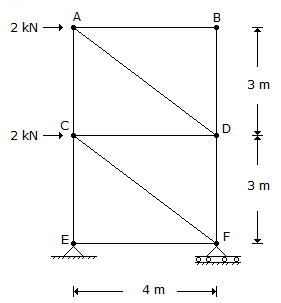A.
 1.5 kN (tension)B.
 4.5 kN (tension)C.
 1.5 kN (compression)D.
 4.5 kN (compression)2.

For designing end bearing piles of square cross-section in clays having average unconfmed compressive strength of 6 t/m2, the net ultimate bearing capacity may be taken as

A.
 15 t/m2B.
 18 t/m2C.
 20 t/m2D.
 27 t/m23.

Timber can be made more fire resistant by

A.
 dipping and steeping processB.
 hot and cold open tank treatmentC.
 charringD.
 Sir Abel's process4.

In a V-notch, an error of 1% in the measurement of head would constitute in the discharge measurement, an error of

A.
 1.0%B.
 1.5%C.
 2.0%D.
 2.5%5.

A fixed beam made of steel is shown in the figure. At collapse, the value of load P will be equal to :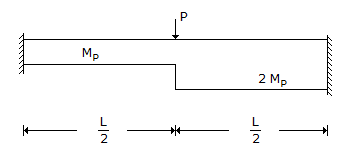A.
 10 Mp/LB.
 12 Mp/LC.
 16 Mp/LD.
 20 Mp/L6.

Consider the following statements :
The use of relatively weak motor
1. will accommodate movements due to loads, cracking if any and will be distributed as thin hair cracks which are less noticeable or harmful.
2. will result in reduction of stresses due to differential expansion of masonry units.
Of these statements :

A.
 1 alone is correctB.
 2 alone is correctC.
 both 1 and 2 are correctD.
 neither 1 nor 1 is correct7.

What does high COD to BOD ratio of an organic pollutant represent ?

A.
 High biodegradability of the pollutantB.
 Low biodegradability of the pollutantC.
 Presence of free oxygen for aerobic decompositionD.
 Presence of toxic material in the pollutants8.

A turn-table on railways is used for

A.
 preventing the lateral movement of wheelsB.
 reversing the direction of the engineC.
 reducing the damage to the railsD.
 reducing the accidents9.

Consider the following statements regarding settlement of foundations :
1. Differential settlement of foundation leads to structural damage to the superstructure.
2. In non-cohesive soils, the major component of settlement is due to consolidation.
3. Lowering of ground water table contributes of settlement of foundations.
Of these statements :

A.
 1 and 2 are correctB.
 1 and 3 are correctC.
 2 and 3 are correctD.
 1, 2 and 3 are correct10.

Ruling gradient on highways as per IRC in plane terrain is

A.
 1 in 30B.
 1 in 60C.
 1 in 100D.
 1 in 20011.

Consider the following statements :
1. Welded structures are usually lighter than riveted structures.
2. Welding allows the arrangement of structural components in such a manner that the joint provides maximum efficiency.
3. During welding, the members do not get distorted.
Of these statements :

A.
 1, 2 and 3 correctB.
 1 and 2 are correctC.
 2 and 3 are correctD.
 1 and 3 are correct12.

The approximate ratio of strength of 15 cm x 30 cm concrete cylinder to that of 15 cm cube of the same concrete is

A.
 1.25B.
 1C.
 0.85D.
 0.513.

Which of the following pairs is/are correctly matched ?
1. Eutrophication.... Nutrient accumulation leading to ecosystem change occurring in impounded water.
2. Autotrophism.... Utilization, rearrangement and decomposition of complex materials predominate.
3. Heterotrophism....Predominance of fixation of light energy, use of simple inorganic substances and built-up complex substances.
Select the correct answer from the codes given below :

A.
 1, 2 and 3B.
 1 aloneC.
 2 and 3D.
 1 and 314.

Which one of the following gives the number of gate position in an airport ?

A.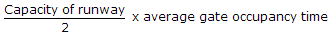B.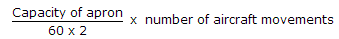C.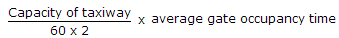D.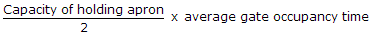15.

The entry of foul smelling gases into the house coming from the sewers can be prevented by

A.
 providing water seals for all the fixturesB.
 providing water seals for all the fixtures and a vent pipe in the plumbing systemC.
 providing sufficient vent pipes in the plumbing systemD.
 exhaust fans16.

Which one of the following can be a set of velocity components of a two-dimensional flow ?

A.
 u = x + y and v = x2 + y2B.
 u = x + y and v = x - yC.
 u = xy and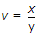D.
 u = x2 + y2 and v = x2 - y217.

For complete hydration of cement the W/C ratio needed is

A.
 less than 0.25B.
 more than 0.25 but less than 0.35C.
 more than 0.35 but less than 0.45D.
 more than 0.45 but less than 0.6018.

A simply supported beam of span l and flexural rigidity EI, carries a unit point load at its centre. The strain energy in the beam due to bending is

A.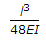B.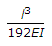C.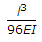D.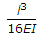19.

A soil sample is having a specific gravity of 2.60 and a void ratio of 0.78. The water content in percentage required to fully saturate the soil at the void ratio would be

A.
 10B.
 30C.
 50D.
 7020.

If a soil sample of weight 0.18 kg having a volume of 10-4 m3 and dry unit wt. of 1600 kg/m3 is mixed with 0.02 kg of water then the water content in the sample will be

A.
 30%B.
 25%C.
 20%D.
 15%